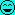# Impact force in rotary motion

• FEAnalyst

#### FEAnalyst

TL;DR Summary
How can I calculate impact force when impactor is in uniform circular motion and then hits the target?
Hi,

how can I calculate the impact force in case when some object is in uniform circular motion and then suddenly hits the target. For example consider lawn mower's blade hitting stone or drum woodchipper's blade hitting branch. Both blades are already moving at full speed when they hit the target. Which equations should I use? Formulas for tangent force in rotary motion do not seem applicable here. The simplest equations for impact force are ##F=m \cdot a## or ##F=\frac{m \Delta v}{\Delta t}##. But what value should I use for acceleration, ##\Delta v## and ##\Delta t## since the blade is already running at full speed? Do I have to know the decceleration occurring when the blade slows down after impact?

Do I have to know the decceleration occurring when the blade slows down after impact?

Yes. It's finding that deceleration (equivalently, finding the deceleration time) that's tricky. It strongly depends on the materials of both objects colliding. When the stress caused by collision is significantly below the yield point of either object, you can use Hooke's law to approximate the time it takes for the impacting object to "bounce" back. Both examples you gave don't satisfy this criterion. I would suggest that this is a problem best left to measurement.

Of course accurate solution would be very hard to get analytically (I can always use dynamic FEA to simulate this event and obtain very good results but that’s not the point here). However, there should be a way to get at least some rough estimate from hand calculations. Maybe I should use one or more of the conservation laws but I’m not sure which one to choose. I guess that someone already performed such calculations and yet I can’t find them anywhere.

Maybe I should use one or more of the conservation laws but I’m not sure which one to choose. I guess that someone already performed such calculations and yet I can’t find them anywhere.
You need more data than you have, and I don't know how to guesstimate it. A time resolved load cell or a high speed visual record would give a good estimate. Without that you are just spitting at it. You do know the energy "used up" but the forces depend upon the time course of the collision.

Wood chipping is roughly a constant force while the blade is in the cut. Cutting force in wood is approximately constant with speed. It's difficult to calculate the cutting force because it is a function of wood density, moisture content, cutting depth, blade geometry, cutting direction relative to wood grain, and blade sharpness. Much easier to measure angular acceleration, and calculate cutting force from acceleration and inertia. From that, torque is force times radius, and acceleration is torque divided by inertia. Or measure total power, and calculate cutting force from number of cuts and length of each cut.

A lawn mower blade hitting a rock is more complicated because the blade can bend or not bend, the rock can break up, go flying, or stay locked in place. Here again, the easiest solution is to measure angular acceleration and calculate force. You may be able estimate impact force by calculating the force needed to get observed blade damage.

•...you don't say